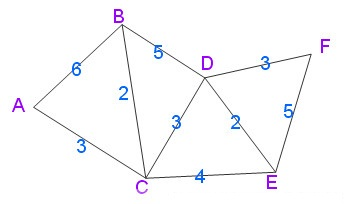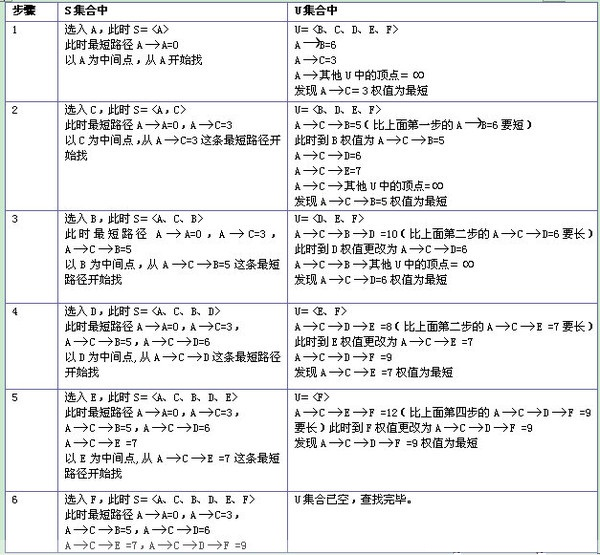•5星
33.77MB goodxianping 2021-09-17 15:03:25
•5星
6.13MB qq_30425067 2021-06-11 15:53:17
•5星
11.8MB guoruibin123 2021-05-21 15:45:32
•5星
3.07MB weixin_57058298 2021-08-12 15:36:06
•5星
220KB weixin_48465965 2021-08-07 15:58:07
•5星
206.62MB u013729183 2021-08-14 08:53:28
•5星
1.67MB Johnho130 2021-05-29 22:33:51
•5星
114KB qq_17556735 2021-03-14 10:30:14
• 最短距离聚类的matlab实现说明：正文中命令部分可以直接在Matlab中运行，作者(Yangfd09)在MATLAB R2009a(7.8.0.347)中运行通过%最短距离聚类%说明：此程序的优点在于每一步都是自己编写的，很少用matlab现成的指令，...

最短距离聚类的matlab实现

说明：正文中命令部分可以直接在Matlab中运行，作者(Yangfd09)在

MATLAB R2009a(7.8.0.347)中运行通过

%最短距离聚类

%说明：此程序的优点在于每一步都是自己编写的，很少用matlab现成的指令，

%所以更适合于初学者，有助于理解各种标准化方法和距离计算方法。

%程序包含了极差标准化(两种方法)、中心化、标准差标准化、总和标准化和极大值标准化等标准化方法，

%以及绝对值距离、欧氏距离、明科夫斯基距离和切比雪夫距离等距离计算方法。

%==========================>>导入数据<<============================== %变量存放在data.mat中，变量名为test

%数据要求：m行n列，m为要素个数，n为区域个数

testdata=test;

%============================>>标准化<<=============================== %变量初始化，m用来寻找每行的最大值，n找最小值，s记录每行数据的和

[M,N]=size(testdata);m=zeros(1,M);n=9999*ones(1,M);s=zeros(1,M);eq=zeros(1,M);

%为m、n和s赋值

for i=1:M

for j=1:N

if testdata(i,j)>=m(i)

m(i)=testdata(i,j);

end

if testdata(i,j)<=n(i)

n(i)=testdata(i,j);

end

s(i)=s(i)+testdata(i,j);

end

eq(i)=s(i)/N;

end

%sigma0是离差平方和，sigma是标准差

sigma0=zeros(M);

for i=1:M

for j=1:N

sigma0(i)=sigma0(i)+(testdata(i,j)-eq(i))^2;

end

end

sigma=sqrt(sigma0/N);

jicha=m-n;

%极差标准化(两种方法)、中心化、标准差标准化、总和标准化和极大值标准化。

展开全文weixin_39581896 2021-04-18 05:33:22
• package com.sage.dss.util;import java.math.BigDecimal;...* 修改了参数，解除对高德 API 的依赖。*/public class MapUtil {/*** 此方法与高德API distance方法算法一致* 计算两点间的距离，单位：米*/publi...

package com.sage.dss.util;

import java.math.BigDecimal;

/**

* 提取自 AMapUtils(版本 com.amap.api:map2d:2.9.1)。

* 修改了参数，解除对高德 API 的依赖。

*/

public class MapUtil {

/**

* 此方法与高德API  distance方法算法一致

* 计算两点间的距离，单位：米

*/

public static BigDecimal calculateLineDistance(double x1, double y1, double x2, double y2) {

double lon1 = (Math.PI / 180) * x1;

double lon2 = (Math.PI / 180) * x2;

double lat1 = (Math.PI / 180) * y1;

double lat2 = (Math.PI / 180) * y2;

if (lon2 == lon1) {

return new BigDecimal(0);

}

// 地球半径

double R = 6378;

// 两点间距离 km，如果想要米的话，结果*1000就可以了

double d = Math.acos(Math.sin(lat1) * Math.sin(lat2) + Math.cos(lat1) * Math.cos(lat2) * Math.cos(lon2 - lon1)) * R;

return new BigDecimal(d);

}

double PointToSegDist(double x, double y, double x1, double y1, double x2, double y2){

double cross = (x2 - x1) * (x - x1) + (y2 - y1) * (y - y1);

if (cross <= 0){

return calculateLineDistance(x, y, x1, y1).doubleValue();

}

double d2 = (x2 - x1) * (x2 - x1) + (y2 - y1) * (y2 - y1);

if (cross >= d2){

return calculateLineDistance(x, y, x2, y2).doubleValue();

}

BigDecimal l1 = calculateLineDistance(x, y, x1, y1);

BigDecimal l2 = calculateLineDistance(x, y, x2, y2);

BigDecimal l12 = calculateLineDistance(x1, y1, x2, y2);

double s = Math.sqrt(l.multiply(l.subtract(l12).multiply(l.subtract(l1).multiply(l.subtract(l2)))).doubleValue());

return new BigDecimal(s).multiply(new BigDecimal(2)).divide(l12, 6).doubleValue();

}

}

展开全文weixin_39618456 2021-04-13 11:31:49
• 点到线段的最短距离算法 算法

点到线段的最短距离算法1. 经典算法2. 面积算法3. 矢量算法 Reference: 点到线段的最短距离算法 点到线段最短距离的运算与点到直线的最短距离的运算二者之间存在一定的差别，即求点到线段最短距离时需要考虑参考点...

点到线段的最短距离算法

Reference:

1. 点到线段的最短距离算法

点到线段最短距离的运算与点到直线的最短距离的运算二者之间存在一定的差别，即求点到线段最短距离时需要考虑参考点在沿线段方向的投影点是否在线段上，若在线段上才可采用点到直线距离公式，如下图所示，

• 左边的最短距离为点 P P 与其在线段 A B AB 上投影 C C 之间的线段 P C PC
• 右边的最短距离为点 P P 与端点 B B A A 所构成的线段 P B PB P A PA具体算法主要有以下三种：

1. 经典算法

该算法直接用高中时所学习到的解析几何知识对点到线段的距离进行求解。其基本思想是先判断点在线段端点、点在线上等等的特殊情况，逐步的由特殊到一般，当忽略点在线段上的特殊情况时，判断点到线段方向的垂线是否落在线段上的方法是通过比较横纵坐标的方式来判断，最后把不同的判断情况用不同的几何方式来进行处理计算得出结果。

由上面叙述的基本思路可以知道这种算法虽然很容易理解和接受，但从算法的实用性的角度分析还是有很大的缺点的，首先是算法复杂，计算量巨大，大量的比较判断、距离计算、角度计算等等，实际应用中往往是需要求由大量线段组成的折线到某点的最短距离，如此用这样的算法计算量是不能想象的。其次经典算法中使用的一些简化运算的函数不利于语言的重新包装，如果想换编程语言的话，就比较麻烦了。

2. 面积算法

该方法主要是先判断投影点是否在线段上，投影点在线段延长线上时，最短距离长度为点到端点的线段长度；当投影点在线段上时，先使用海伦公式计算三角形面积，再计算出三角形的高，即为最短距离。

运用面积算法求解点到线段最短距离思路很清晰，也很容易理解。从效率方面考虑，比如需要多次计算平方、根号，这对于大量数据进行运算是负担很重的。求面积就必须把三条边长全部求出，并且用到的海伦公式也需要进行开方运算，计算过程显得繁琐。

3. 矢量算法

矢量算法过程清晰，如果具有一定的空间几何基础，则是解决此类问题时应优先考虑的方法。当需要计算的数据量很大时，这种方式优势明显。

由于矢量具有方向性，故一些方向的判断直接根据其正负号就可以得知，使得其中的一些问题得以很简单的解决。

用此方法考虑，我们只需要找到向量 A P → \overrightarrow{AP} A B → \overrightarrow{AB} 方向上的投影，具体如下：A C → = ( A P → ⋅ A B → ) ∣ A B → ∣ 2 A B → = ( A P → ⋅ A B → ) ∣ A B → ∣ ⋅ A B → ∣ A B → ∣ \overrightarrow{A C}=\frac{(\overrightarrow{A P} \cdot \overrightarrow{A B})}{|\overrightarrow{A B}|^2} \overrightarrow{A B}=\frac{(\overrightarrow{A P} \cdot \overrightarrow{A B})}{|\overrightarrow{A B}|} \cdot \frac{\overrightarrow{A B}}{|\overrightarrow{A B}|}

上面的 A B → A B → \frac{\overrightarrow{A B}}{\overrightarrow{A B}} A B → \overrightarrow{A B} 方向上的单位向量，其意义是给所求向量确定方向。 A P → ⋅ A B → \overrightarrow{A P} \cdot \overrightarrow{A B} 是两个向量的内积，且 A P → ⋅ A B → = ∣ A P → ∣ ∣ A B → ∣ cos ⁡ ( θ ) \overrightarrow{A P} \cdot \overrightarrow{A B} = |\overrightarrow{A P}| |\overrightarrow{A B}|\cos(\theta) ，其中 θ \theta 为向量 A P → \overrightarrow{A P} A B → \overrightarrow{A B} 之间的夹角。 ∣ A B → ∣ |\overrightarrow{A B}| 是向量长度。

那么 ( A P → ⋅ A B → ) ∣ A B → ∣ = ∣ A P → ∣ ∣ A B → ∣ cos ⁡ θ ∣ A B → ∣ = ∣ A P → ∣ cos ⁡ θ \frac{(\overrightarrow{A P} \cdot \overrightarrow{A B})}{|\overrightarrow{A B}|}=\frac{|\overrightarrow{A P}||\overrightarrow{A B}| \cos \theta}{|\overrightarrow{A B}|}=|\overrightarrow{A P}| \cos \theta 即为上图中线段 A C AC 的长度值，不带有方向性。此数值加上方向 A B → A B → \frac{\overrightarrow{A B}}{\overrightarrow{A B}} 即可构成：有大小和方向的新向量 A C → \overrightarrow{A C} ，即为 A P → \overrightarrow{A P} A B → \overrightarrow{A B} 方向上的投影向量， C C 为投影点。

根据得到的 r = ( A P → ⋅ A B → ) ∣ A B → ∣ 2 r=\frac{(\overrightarrow{A P} \cdot \overrightarrow{A B})}{|\overrightarrow{A B}|^{2}} ，由向量的方向性可知：

• 如果情况是上图 ( a ) (a) 所示，那么 0 < r < 1 0<r<1
• 如果情况是上图 ( b ) (b) 所示，那么 r > = 1 r>=1
• 如果情况是上图 ( c ) (c) 所示，那么 r < = 0 r<=0

特殊情况如点在线段上点在端点点在线段延长线上等等情况全部适用于此公式，只是作为特殊情况出现，无需另做讨论。这也是适量算法思想的优势所在。

故根据 r r 值的不同，最短距离 d d 可以计算为：
d = { ∣ A P → ∣  if  r ≤ 0 ∣ B P → ∣  if  r ≥ 1 ∣ C P → ∣  otherwise  d=\left\{\begin{array}{l}|\overrightarrow{A P}| \text { if } r \leq 0 \\ |\overrightarrow{B P}| \text { if } r \geq 1 \\ |\overrightarrow{C P} | \text { otherwise }\end{array}\right.

C#代码为：

double PointToSegDist(double x, double y, double x1, double y1, double x2, double y2)
{
double cross = (x2 - x1) * (x - x1) + (y2 - y1) * (y - y1);
if (cross <= 0) return Math.Sqrt((x - x1) * (x - x1) + (y - y1) * (y - y1));

double d2 = (x2 - x1) * (x2 - x1) + (y2 - y1) * (y2 - y1);
if (cross >= d2) return Math.Sqrt((x - x2) * (x - x2) + (y - y2) * (y - y2));

double r = cross / d2;
double px = x1 + (x2 - x1) * r;
double py = y1 + (y2 - y1) * r;
return Math.Sqrt((x - px) * (x - px) + (y - py) * (y - py))
}

展开全文qq_28087491 2021-07-30 10:33:17
• JAVA实现最短距离算法之迪杰斯特拉算法 java 迪杰斯特拉

最短路径问题是图论研究中的一个经典的算法问题，旨在寻找图中两个节点之间的最短路径，常用的算法有Dijkstra算法、SPFA算法\Bellman-Ford算法、Floyd算法\Floyd-Warshall算法、Johnson算法等，这篇博客将重点介绍...

最短路径问题是图论研究中的一个经典的算法问题，旨在寻找图中两个节点之间的最短路径，最常用的算法有Dijkstra算法、SPFA算法\Bellman-Ford算法、Floyd算法\Floyd-Warshall算法、Johnson算法等，这篇博客将重点介绍Dijkstra算法。

迪杰斯特拉算法

迪杰斯特拉算法是由荷兰计算机科学家狄克斯特拉于1959 年提出的，因此又叫狄克斯特拉算法。是从一个顶点到其余各顶点的最短路径算法，解决的是有向图中最短路径问题。迪杰斯特拉算法主要特点是以起始点为中心向外层层扩展，直到扩展到终点为止。具体的计算规则我们可以通过下图进行查看。通过这幅图(如果图片无法正确显示，请通过百度百科查看)我们可以简单的理解迪杰斯特拉算法算法的基础思路，下面我们就通过Java来实现这个算法。

先给出一个无向图用Dijkstra算法找出以A为起点的单源最短路径步骤如下算法实现

在具体的实现之前，我们先有一个基础的约定，就是途中的每一个节点我们都用正整数进行编码，相邻两点之间的距离是正整数，图中两个直接相邻两点的距离我们保存到map中，也就是求最短距离我们需要实现这样的一个方法：

public MinStep getMinStep(int start, int end, final HashMap> stepLength);

第一个参数是起始节点的编号，第二个参数是终点节点的编号，第三个参数是图中直接相邻两个节点的距离组成的map。关于第三个参数，会在后面做详细的介绍。这里我们定义一个接口，用于计算两点之间的最短路径，具体如下：

/**

*@Description:

*/

package com.lulei.distance;

import java.util.HashMap;

import com.lulei.distance.bean.MinStep;

public interface Distance {

public static final MinStep UNREACHABLE = new MinStep(false, -1);

/**

* @param start

* @param stepLength

* @Author:lulei

* @Description: 起点到终点的最短路径

*/

public MinStep getMinStep(int start, int end, final HashMap> stepLength);

}

一、方法返回值介绍

上面方法的返回值是我们自定义的一个数据类型，下面我们通过代码来看其具体的数据结构：

/**

*@Description:

*/

package com.lulei.distance.bean;

import java.util.List;

public class MinStep {

private boolean reachable;//是否可达

private int minStep;//最短步长

private List step;//最短路径

public MinStep() {

}

public MinStep(boolean reachable, int minStep) {

this.reachable = reachable;

this.minStep = minStep;

}

public boolean isReachable() {

return reachable;

}

public void setReachable(boolean reachable) {

this.reachable = reachable;

}

public int getMinStep() {

return minStep;

}

public void setMinStep(int minStep) {

this.minStep = minStep;

}

public List getStep() {

return step;

}

public void setStep(List step) {

this.step = step;

}

}

其中最短路径的那个List数组保存了从起点到终点最短路径所经历的节点。

二、每一个节点的最优前一节点

在迪杰斯特拉算法中我们需要保存从起点开始到每一个节点最短步长，这也是图中需要比较得出的步长，同时我们还需要存储该步长下的前一个节点是哪个，这样我们就可以通过终点一个一个往前推到起点，这样就出来了完整的最优路径。

/**

*@Description:

*/

package com.lulei.distance.bean;

public class PreNode {

private int preNodeNum;// 最优 前一个节点

private int nodeStep;// 最小步长

public PreNode(int preNodeNum, int nodeStep) {

this.preNodeNum = preNodeNum;

this.nodeStep = nodeStep;

}

public int getPreNodeNum() {

return preNodeNum;

}

public void setPreNodeNum(int preNodeNum) {

this.preNodeNum = preNodeNum;

}

public int getNodeStep() {

return nodeStep;

}

public void setNodeStep(int nodeStep) {

this.nodeStep = nodeStep;

}

}

三、迪杰斯特拉算法计算过程中需要关注的变量

从介绍迪杰斯特拉算法的图中，我们知道在计算的过程中我们需要保存起点到各个节点的最短距离、已经计算过的节点、下次需要计算节点队列和图中相邻两个节点的距离。我们通过代码来看下具体的定义：

//key1节点编号，key2节点编号，value为key1到key2的步长

private HashMap> stepLength;

//非独立节点个数

private int nodeNum;

//移除节点

private HashSet outNode;

//起点到各点的步长，key为目的节点，value为到目的节点的步长

private HashMap nodeStep;

//下一次计算的节点

//起点、终点

private int startNode;

private int endNode;

我们这里看下stepLength这个属性，它保存了图中相邻两个节点之间的距离，比如key1=1;key2=3;value=9;这代表的意义就是从节点1到节点3的距离是9。通过这样的存储，我们就需要把图中每两个相邻的点保存到这个类型的map中。

四、属性初始化

在开始计算之前，我们需要对这些属性进行初始化，具体如下：

private void initProperty(int start, int end) {

outNode = new HashSet();

nodeStep = new HashMap();

startNode = start;

endNode = end;

}

这一步我们需要把起点添加到下一次需要计算的节点队列中。

五、迪杰斯特拉算法

这一步也就是迪杰斯特拉算法的核心部分，在计算的过程中，我们需要进行如下步骤：

1)判断是否达到终止条件，如果达到终止条件，结束本次算法，如果没有达到，执行下一步；(终止条件：下一次需要计算的节点队列没有数据或已经计算过的节点数等于节点总数)

2)获取下一次计算的节点A；

3)从起点到各节点之间的最短距离map中获取到达A点的最小距离L；

4)获取A节点的可达节点B，计算从起点先到A再到B是否优于已有的其他方式到B，如果优于，则更新B节点，否则不更新；

5)判断B是否是已经移除的节点，如果不是移除的节点，把B添加到下一次需要计算的节点队列中，否则不做操作；

6)判断A节点是否还有除B以外的其他节点，如果有，执行第4)步，否则执行下一步；

7)将A节点从下一次需要计算的节点中移除添加到已经计算过的节点中；

8)执行第一步。

我们来看下具体的代码实现：

private void step() {

if (nextNode == null || nextNode.size()

return;

}

if (outNode.size() == nodeNum) {

return;

}

//获取下一个计算节点

int start = nextNode.removeFirst();

//到达该节点的最小距离

int step = 0;

if (nodeStep.containsKey(start)) {

step = nodeStep.get(start).getNodeStep();

}

//获取该节点可达节点

HashMap nextStep = stepLength.get(start);

Iterator> iter = nextStep.entrySet().iterator();

while (iter.hasNext()) {

Entry entry = iter.next();

Integer key = entry.getKey();

//如果是起点到起点，不计算之间的步长

if (key == startNode) {

continue;

}

//起点到可达节点的距离

Integer value = entry.getValue() + step;

if ((!nextNode.contains(key)) && (!outNode.contains(key))) {

}

if (nodeStep.containsKey(key)) {

if (value

nodeStep.put(key, new PreNode(start, value));

}

} else {

nodeStep.put(key, new PreNode(start, value));

}

}

//将该节点移除

//计算下一个节点

step();

}

六、组装最短路径返回结果

通过前面的计算，已经计算出了起点到各个节点的最短路径，下面就需要组装起点到终点的最短路径，在计算最短距离下的路径方式，我们需要从终点依次往前推，即到达终点最短距离下的前一个节点是A，到达A节点最短距离下的前一节点是B，直到找到起点终止。

private MinStep changeToMinStep() {

MinStep minStep = new MinStep();

minStep.setMinStep(nodeStep.get(endNode).getNodeStep());

minStep.setReachable(true);

minStep.setStep(step);

int nodeNum = endNode;

while (nodeStep.containsKey(nodeNum)) {

int node = nodeStep.get(nodeNum).getPreNodeNum();

nodeNum = node;

}

return minStep;

}

七、接口定义方法实现

public MinStep getMinStep(int start, int end, final HashMap> stepLength) {

this.stepLength = stepLength;

this.nodeNum = this.stepLength != null ? this.stepLength.size() : 0;

//起点、终点不在目标节点内，返回不可达

if (this.stepLength == null || (!this.stepLength.containsKey(start)) || (!this.stepLength.containsKey(end))) {

return UNREACHABLE;

}

initProperty(start, end);

step();

if (nodeStep.containsKey(end)) {

return changeToMinStep();

}

return UNREACHABLE;

}

八、迪杰斯特拉算法完整代码

/**

*@Description:

*/

package com.lulei.distance.dijkstra;

import java.util.HashMap;

import java.util.HashSet;

import java.util.Iterator;

import java.util.Map.Entry;

import com.lulei.distance.Distance;

import com.lulei.distance.bean.MinStep;

import com.lulei.distance.bean.PreNode;

public class DistanceDijkstraImpl implements Distance{

//key1节点编号，key2节点编号，value为key1到key2的步长

private HashMap> stepLength;

//非独立节点个数

private int nodeNum;

//移除节点

private HashSet outNode;

//起点到各点的步长，key为目的节点，value为到目的节点的步长

private HashMap nodeStep;

//下一次计算的节点

//起点、终点

private int startNode;

private int endNode;

/**

* @param start

* @param end

* @param stepLength

* @return

* @Author:lulei

* @Description: start 到 end 的最短距离

*/

public MinStep getMinStep(int start, int end, final HashMap> stepLength) {

this.stepLength = stepLength;

this.nodeNum = this.stepLength != null ? this.stepLength.size() : 0;

//起点、终点不在目标节点内，返回不可达

if (this.stepLength == null || (!this.stepLength.containsKey(start)) || (!this.stepLength.containsKey(end))) {

return UNREACHABLE;

}

initProperty(start, end);

step();

if (nodeStep.containsKey(end)) {

return changeToMinStep();

}

return UNREACHABLE;

}

/**

* 返回最短距离以及路径

*/

private MinStep changeToMinStep() {

MinStep minStep = new MinStep();

minStep.setMinStep(nodeStep.get(endNode).getNodeStep());

minStep.setReachable(true);

minStep.setStep(step);

int nodeNum = endNode;

while (nodeStep.containsKey(nodeNum)) {

int node = nodeStep.get(nodeNum).getPreNodeNum();

nodeNum = node;

}

return minStep;

}

/**

* @param start

* @Author:lulei

* @Description: 初始化属性

*/

private void initProperty(int start, int end) {

outNode = new HashSet();

nodeStep = new HashMap();

startNode = start;

endNode = end;

}

/**

* @param end

* @Author:lulei

* @Description:

*/

private void step() {

if (nextNode == null || nextNode.size()

return;

}

if (outNode.size() == nodeNum) {

return;

}

//获取下一个计算节点

int start = nextNode.removeFirst();

//到达该节点的最小距离

int step = 0;

if (nodeStep.containsKey(start)) {

step = nodeStep.get(start).getNodeStep();

}

//获取该节点可达节点

HashMap nextStep = stepLength.get(start);

Iterator> iter = nextStep.entrySet().iterator();

while (iter.hasNext()) {

Entry entry = iter.next();

Integer key = entry.getKey();

//如果是起点到起点，不计算之间的步长

if (key == startNode) {

continue;

}

//起点到可达节点的距离

Integer value = entry.getValue() + step;

if ((!nextNode.contains(key)) && (!outNode.contains(key))) {

}

if (nodeStep.containsKey(key)) {

if (value

nodeStep.put(key, new PreNode(start, value));

}

} else {

nodeStep.put(key, new PreNode(start, value));

}

}

//将该节点移除

//计算下一个节点

step();

}

}

代码测试

对于上述代码的测试，我们还是使用我们事例图形中的例子，计算从节点1到节点5的最短距离。

/**

*@Description:

*/

package com.lulei.distance.test;

import java.util.HashMap;

import com.lulei.distance.Distance;

import com.lulei.distance.bean.MinStep;

import com.lulei.distance.dijkstra.DistanceDijkstraImpl;

import com.lulei.util.JsonUtil;

public class DistanceTest {

public static void main(String[] args) {

// TODO Auto-generated method stub

HashMap> stepLength = new HashMap>();

HashMap step1 = new HashMap();

stepLength.put(1, step1);

step1.put(6, 14);

step1.put(3, 9);

step1.put(2, 7);

HashMap step2 = new HashMap();

stepLength.put(2, step2);

step2.put(1, 7);

step2.put(3, 10);

step2.put(4, 15);

HashMap step3 = new HashMap();

stepLength.put(3, step3);

step3.put(1, 9);

step3.put(2, 10);

step3.put(4, 11);

step3.put(6, 2);

HashMap step4 = new HashMap();

stepLength.put(4, step4);

step4.put(2, 15);

step4.put(5, 5);

step4.put(3, 11);

HashMap step5 = new HashMap();

stepLength.put(5, step5);

step5.put(6, 9);

step5.put(4, 5);

HashMap step6 = new HashMap();

stepLength.put(6, step6);

step6.put(1, 14);

step6.put(5, 9);

step6.put(3, 2);

Distance distance = new DistanceDijkstraImpl();

MinStep step = distance.getMinStep(1, 5, stepLength);

System.out.println(JsonUtil.parseJson(step));

}

}

这里组装相邻两个节点之间的距离用了大量的代码，我们看下输出结果：

{"reachable":true,"minStep":20,"step":[1,3,6,5]}

最后的思考

最短路径算法在现实生活中其实有很多的用处，比如迷宫解法、路径规划、路由寻址等等，这些问题看似很复杂，其实只需要做对应的转化后还是可以用最基础的算法解决的。

展开全文weixin_39648824 2021-02-12 19:47:45
• weixin_39664998 2021-03-11 17:03:12
• weixin_28481133 2021-03-13 12:34:37
• weixin_39871649 2021-01-17 19:16:39
• it_xiangqiang 2021-03-29 09:17:58
• 聚类分析—最大最小距离算法实现 聚类 算法

• weixin_30748871 2020-12-29 04:23:13
• weixin_32183427 2021-03-13 12:34:30
• 最大最小距离法 机器学习

mu_to 2021-10-14 11:37:42
• weixin_39738774 2020-12-19 14:15:47
• qq_43406565 2021-04-03 15:00:05
• 聚类算法-最大最小距离算法 算法

GRQHHH 2021-10-13 22:33:50
• weixin_39939303 2020-12-19 14:15:56
• 两点之间最短距离：贪心算法 （DIJKSTRA算法） 贪心算法 算法 c语言

weixin_56977307 2021-12-15 21:45:46
• weixin_39557576 2020-12-19 14:16:21
• weixin_39637589 2020-12-19 14:15:46
• 【Python】实现最大最小距离算法 聚类 算法 python 机器学习 数据挖掘

muzihuaner 2021-09-30 06:09:13
• 数学建模-蚁群算法-最短距离（优化模型） 数学 数学建模 算法

ASHIYI66 2021-08-01 11:52:14
• weixin_39999781 2020-12-19 14:15:49
• 【星球两点距离】——最短距离计算理论和代码实现 python 编程语言

BlackValley 2021-07-04 22:54:46
• 系统聚类法的SAS编程与R编程（代码） 聚类算法

qq_44876504 2021-06-27 10:34:55
• SPFA算法：找单源点最短距离 算法 数据结构

qq_48988844 2021-09-24 20:54:40
• 最短路径-任意两点间最短距离-Floyd算法的matlab实现（详细教程） matlab 算法 动态规划

qq_61123461 2021-09-01 20:14:18
• weixin_39620662 2020-12-19 14:15:47
• weixin_39633452 2020-12-19 14:15:08
• weixin_39955953 2020-12-23 01:11:43...